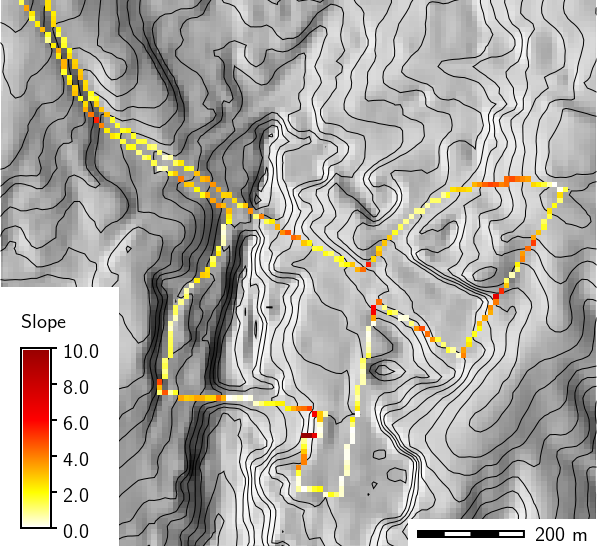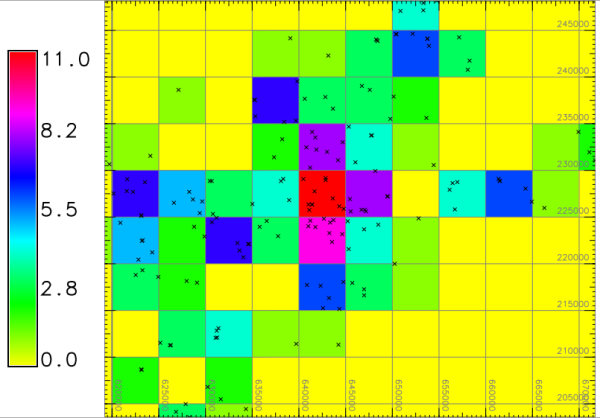Note: This document is for an older version of GRASS GIS that will be discontinued soon. You should upgrade, and read the current manual page.

## NAME

v.to.rast - Converts (rasterize) a vector map into a raster map.

## KEYWORDS

vector, conversion, raster, rasterization

## SYNOPSIS

v.to.rast
v.to.rast --help
v.to.rast [-d] input=name [layer=string] [type=string[,string,...]] [cats=range] [where=sql_query] output=name use=string [attribute_column=name] [rgb_column=name] [label_column=name] [value=float] [memory=memory in MB] [--overwrite] [--help] [--verbose] [--quiet] [--ui]

### Flags:

-d
Create densified lines (default: thin lines)
All cells touched by the line will be set, not only those on the render path
--overwrite
Allow output files to overwrite existing files
--help
Print usage summary
--verbose
Verbose module output
--quiet
Quiet module output
--ui
Force launching GUI dialog

### Parameters:

input=name [required]
Name of input vector map
Or data source for direct OGR access
layer=string
Layer number or name
Vector features can have category values in different layers. This number determines which layer to use. When used with direct OGR access this is the layer name.
Default: 1
type=string[,string,...]
Input feature type
Options: point, line, boundary, centroid, area
Default: point,line,area
cats=range
Category values
Example: 1,3,7-9,13
where=sql_query
WHERE conditions of SQL statement without 'where' keyword
Example: income < 1000 and population >= 10000
output=name [required]
Name for output raster map
use=string [required]
Source of raster values
Options: attr, cat, val, z, dir
attr: read values from attribute table
cat: use category values
val: use value specified by value option
z: use z coordinate (points or contours only)
dir: line direction in degrees CCW from east (lines only)
attribute_column=name
Name of column for 'attr' parameter (data type must be numeric)
rgb_column=name
Name of color definition column (with RRR:GGG:BBB entries)
label_column=name
Name of column used as raster category labels
value=float
Raster value (for use=val)
Default: 1
memory=memory in MB
Maximum memory to be used (in MB)
Cache size for raster rows
Default: 300

## DESCRIPTION

v.to.rast transforms GRASS vector map layers into GRASS raster map layer format. Optionally, attributes can be converted to raster category labels.

## NOTES

In order to avoid unexpected results, the type of vector features should always be specified. The default is to convert all vector features, but if only e.g. areas should be converted use type=area rather than type=point,line,area.

v.to.rast will only affect data in areas lying inside the boundaries of the current geographic region. A grid cell belongs to the area where the grid cell center falls into.

Before running v.to.rast, the user should therefore ensure that the current geographic region is correctly set and that the region resolution is at the desired level.

Either the column parameter or the value parameter must be specified. The use option may be specified alone when using the dir option.

use options are:

• attr - read values from attribute table (default)
• cat - read values from category
• val - use value specified by value option
• z - use z coordinate (points or contours only)
• dir - line direction in degrees counterclockwise from east (lines only)

The column parameter uses an existing column from the vector map database table as the category value in the output raster map. Existing table columns can be shown by using db.describe.

An empty raster map layer will be created if the vector map layer has not been assigned category/attribute labels (e.g., through use of v.category option=add).

Otherwise:

• Labeled areas and/or centroids will produce filled raster coverages with edges that straddle the original area boundary as long as the boundary is NOT labeled.
(Use v.category option=del type=boundary to remove.)
• Labeled lines and boundaries will produce lines of raster cells which touch the original vector line. This tends to be more aggressive than area-only conversions.
• Points and orphaned centroids will be converted into single cells on the resultant raster map.

Line directions are given in degrees counterclockwise from east.

Raster category labels are supported for all of use= except use=z.

The -d flag applies only to lines and boundaries, the default is to set only those cells on the render path (thin line).

Boundaries (usually without categories) can be rasterized with

```v.to.rast type=boundary layer=-1 use=val
```

## EXAMPLES

### Convert a vector map and use column SPEED from attribute table

```db.describe -c table=vect_map

ncols:3
Column 1: CAT
Column 2: SPEED
Column 3: WIDTH
```
```v.to.rast input=vect_map output=raster_map attribute_column=SPEED type=line
```

### Calculate stream directions from a river vector map (Spearfish)

```v.to.rast input=streams output=streamsdir use=dir
```

### Calculate slope along path

Using slope and aspect maps, compute slope along a bus route (use full NC sample dataset):
```g.region raster=elevation -p
r.slope.aspect elevation=elevation slope=slope aspect=aspect

# compute direction of the bus route
v.to.rast input=busroute11 type=line output=busroute11_dir use=dir

# extract steepest slope values and transform them into slope along path
r.mapcalc "route_slope = if(busroute11, slope)"
r.mapcalc "route_slope_dir = abs(atan(tan(slope) * cos(aspect - busroute11_dir)))"
```Slope in degrees along bus route

### Convert a vector polygon map to raster including descriptive labels

In this example, the ZIP code vector map is rasterized (North Carolina sample dataset):
```# rasterize ZIP codes at 50m raster resolution
g.region vector=zipcodes_wake res=50 -ap
# vector to raster conversion, with category labels
v.to.rast input=zipcodes_wake output=myzipcodes use=attr attribute_column="ZIPNUM" label_column="NAME"
```

### Convert vector points to raster with raster cell binning

In this example, the number of schools per raster cell are counted (North Carolina sample dataset):
```g.copy vector=schools_wake,myschools_wake

# set computation region for raster binning
g.region vector=myschools_wake res=5000 -p -a

# add new column for counting
v.db.update myschools_wake column=value value=1

# verify attributes
v.db.select myschools_wake column=cat,value
v.out.ascii input=myschools_wake output=- column=value

# export and import on the fly, use 4th column (value) as input
v.out.ascii input=myschools_wake output=- column=value | r.in.xyz input=- \
z=4 output=schools_wake_aggreg method=sum

d.mon wx0
d.rast schools_wake_aggreg
d.vect schools_wake
d.grid 5000
```Number of schools per raster cell

db.describe, v.category

## AUTHORS

Original code: Michael Shapiro, U.S. Army Construction Engineering Research Laboratory